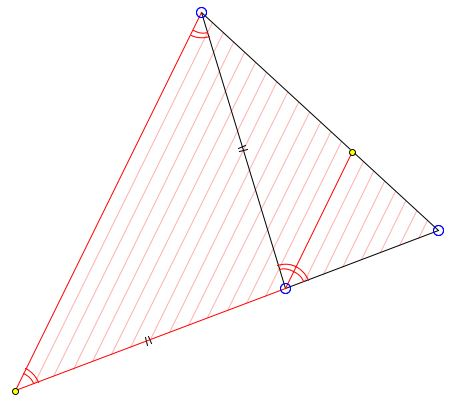# Property of Angle BisectorsWhat is this about? A Mathematical Droodle

### If you are reading this, your browser is not set to run Java applets. Try IE11 or Safari and declare the site https://www.cut-the-knot.org as trusted in the Java setup.What if applet does not run?

ExplanationAngle bisectors divide the opposite side in the ratio of the adjacent sides. More accurately,

If, in ΔABC, AD is an angle bisector of angle A, then

AB/AC = DB/DC.

Note that the same holds also for the external angle bisectors.### Proof

Assume the straight line through C parallel to AD meets AB in E. Then, first of all, ΔAEC is isosceles: AC = AE. This is because

Therefore, AE = AC, and the required proportion follows from the similarity of triangles BEC and BAD. (There is a less standard proof.)

This property of angle bisectors is one way to show that the three angle bisectors in a triangle meet in a point. The result is an immediate consequence of Ceva's theorem.

Last note: the converse theorem holds as a matter of course, because there is only one point on a given segment that divides it in a given ratio. Thus if a point divides the base of a triangle in the ratio equal to the ratio of the sides, it is bound to be the foot of the angle bisector from the apex.### Angle Bisector

• Angle Bisector
• Angle Bisector Theorem
• Angle Bisectors in Ellipse
• Angle Bisectors in Ellipse II
• Angle Bisector in Equilateral Trapezoid
• Angle Bisector in Rectangle
• Property of Angle Bisectors II
• External Angle Bisectors
• Projections on Internal and External Angle Bisectors
• Angle Bisectors On Circumcircle
• Angle Bisectors in a Quadrilateral - Cyclic and Otherwise
• Problem: Angle Bisectors in a Quadrilateral
• Triangle From Angle Bisectors
• Property of Internal Angle Bisector - Hubert Shutrick's PWW
• Angle Bisectors Cross Circumcircle
• For Equality Choose Angle Bisector
•